visual line following
David  [14 posts]
7 years
 hi steven I see the tutorial but I don't understand some function. if i want to check out the defect of the line to give out the signal of  "defect" text, it's use display variable?David  [14 posts] 7 years
 also i don't understand the VBScript in line following in tutorial can somebody can help me, thank you below is the VBScript in line following x = GetVariable("COG_X") y = GetVariable("COG_Y") cx = GetVariable("IMAGE_WIDTH") / 2      why it need to divide by 2 cy = GetVariable("IMAGE_HEIGHT") / 2     why it need to divide by 2 xoff = GetVariable("IMAGE_WIDTH") / 8   why it need to divide by 8 yoff = GetVariable("IMAGE_HEIGHT") / 16  why it need to divide by 16 also x < cx - xoff means? if x < cx - xoff then         if y < cy - yoff then     move = "pivot left"   else     move = "left"   end if elseif x > cx + xoff then   if y < cy - yoff then     move = "pivot right"   else     move = "right"   end if else   if y < cy - yoff then     move = "backup"   else     move = "straight"   end if end if SetVariable "move", moveCarl from United States  [18 posts] 7 years
 David, cx = GetVariable("IMAGE_WIDTH") / 2 Finds the horizontal center of the image. cy = GetVariable("IMAGE_HEIGHT") / 2 Finds the vertical center of the image. xoff = GetVariable("IMAGE_WIDTH") / 8 Finds the sensitivity cutoff for horizontal (left/right) correction. yoff = GetVariable("IMAGE_HEIGHT") / 16 Finds the sensitivity cutoff for vertical (forward/backward) correction. if x < cx - xoff then Determines if we need to go to the left, since it's checking if x (COG_X) is less than the difference between the horizontal center of the image and the sensitivity cutoff for left/right course change. This makes sense if you remember that the zero coordinates (x=0 and y=0) are at the bottom left corner of the image. Display_Variable shows the value of a variable on screen, but it doesn't determine the presence or absence of a defect. I'm unsure of the best way of detecting the defect, STeven is sharper about such things than I am.  :) Cheers, CarlDavid  [14 posts] 7 years
 thank you Carl after seeing your comment i think i understand the programDavid  [14 posts] 7 years
 Also i want to add the detection to detect green light ,yellow light, red light to give out the signal "go", "prepare to go /stop" " stop" respectively. i seen the same post http://www.roborealm.com/forum/index.php?thread_id=1308 that when open it give out the warning how to fix it in uploads image also should i use color detection to detect the light?better? could everyone can help me, thanks a lot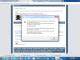David from Hong Kong  [1 posts] 7 years
 it is possible to do? thanks a lotCarl from United States  [18 posts] 7 years
 Firstly for the file - when you acknowledge the error and close that window just do a save.  When you reload it it works fine although you'll want to set  your camera if you're going to test. As for adding the yellow light, it's not particularly difficult, you can simply copy what was done for the red and green lights, and in the RGB_Filter module of the copy change the setting to yellow and the assignment of traffic_signal to your prepare to go /stop.David  [14 posts] 7 years
 thanks a lot but i want to add the if statement to check out if the green light is detected, the outcome will show a text " go" otherwise it will not give out any display i don't understand the if statement show i use setvariable functionDavid  [14 posts] 7 years
 also i want to add the function to calculate the angle it is suitable ? could someone can give me a suggestion. thank you.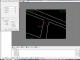Carl from United States  [18 posts] 7 years
 As for the first question, the easiest explaination is the attached robofile. The modules have appropriate comments so it's hopefully fairly obvious. Among the problems with what you tried is that you're comparing a position with a count, which doesn't give a meaningful answer.  The Blob_Filter module is what allowed the earlier version to distinguish between a traffic light and other arbitrary things once color was handled. As to the second question, you have a few problems in your setup. The Calculate_Angle module needs the values produced by the Center_of_Gravity module, so for correct results it should be in the opposite order in the pipeline. The modules in the pipeline execute once per frame from the camera, and do so in the sequence in which you placed them. I can't say what your VBscript program is doing beyond probably calculating move since its content is not exposed, but in the Calculate_Angle module you're using it for both XEnd and YEnd which doesn't make sense either.program.roboDavid  [14 posts] 7 years
 Carl, for the second question, I use the tutorial(visual line following) to add the calculate_angle function MY target is that use the camera to detect the Electrical tape -------------------------------------------------------------------------------------- x = GetVariable("COG_X") y = GetVariable("COG_Y") cx = GetVariable("IMAGE_WIDTH") / 2 cy = GetVariable("IMAGE_HEIGHT") / 2 xoff = GetVariable("IMAGE_WIDTH") / 8 yoff = GetVariable("IMAGE_HEIGHT") / 16 if x < cx - xoff then   if y < cy - yoff then     move = "pivot left"   else     move = "left"   end if elseif x > cx + xoff then   if y < cy - yoff then     move = "pivot right"   else     move = "right"   end if else   if y < cy - yoff then     move = "backward"   else     move = "straight"   end if end if SetVariable "move", move --------------------------------------------------------------- the "move" is from VBScript start point is in "Cog_x"  & "Cog_y"   XEnd and YEnd should select?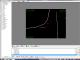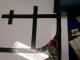program.roboCarl from United States  [18 posts] 7 years
 David, The result in move is not a number, so trying to use it as one doesn't work.  And although CAMERA_PATH may sound promising by name it's not a number either, it's the usb device path of the camera that you are currently connected to. When in doubt as to what a variable really contains Watch_Variables is a convenient way to find out. Since you're trying to get the angle between the camera's center of view and the COG, you'd use half the image dimensions much as you did in the VBscript file. The simplest way is to fill in the fields of the Calculate_Angle module with XEnd being [IMAGE_WIDTH/2] and YEnd being [IMAGE_HEIGHT/2] as shown in the attached photo. Text field expressions are often useful, see http://www.roborealm.com/help/Expressions.php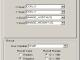This forum thread has been closed due to inactivity (more than 4 months) or number of replies (more than 50 messages). Please start a New Post and enter a new forum thread with the appropriate title.